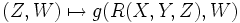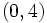# Riemannian curvature space

Let$V$ be a real vector space with a Euclidean inner product. The Riemannian curvature space of$V$ is the space of$(1,3)$-tensors on$V$ of the following description:$R$ is in the Riemannian curvature space if$R(X,Y,Z)$ is alternating in$X$ and$Y$, and further, for any$X,Y$, the map$(Z,W) \mapsto g(R(X,Y,Z),W)$ is alternating in$Z$ and$W$.
By the canonical identification of$(1,3)$-tensors with$(0,4)$-tensors, the Riemannian curvature space can be identified with the symmetric square of the exterior square of the vector space.